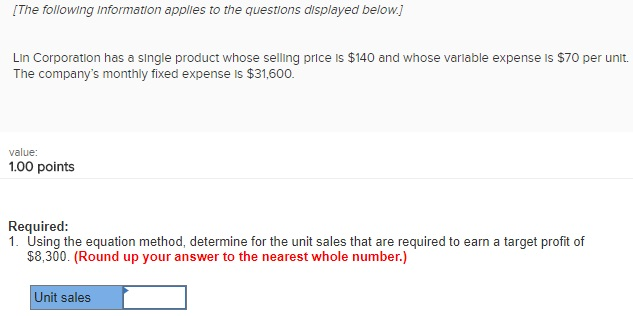# Question & Answer: Lin Corporation has a single product whose selling price is \$140 and whose variable expense is \$70 per u…..Lin Corporation has a single product whose selling price is \$140 and whose variable expense is \$70 per unit. The company’s monthly fixed expense is \$31, 600. Required: Using the equation method, determine for the unit sales that are required to earn a target profit of \$8, 300. (Round up your answer to the nearest whole number.)

Don't use plagiarized sources. Get Your Custom Essay on
Question & Answer: Lin Corporation has a single product whose selling price is \$140 and whose variable expense is \$70 per u…..
GET AN ESSAY WRITTEN FOR YOU FROM AS LOW AS \$13/PAGE

Let units sold be x.

Using the equation method:

Sales=Variable expense+Fixed expense+Target profit

140x=70x+31600+8300

70x=39900.

Hence x=unit sales=(39900/70)=570